# Gerak lurus

### Dari Wikipedia bahasa Indonesia, ensiklopedia bebas

Gerak lurus adalah gerak suatu obyek yang lintasannya berupa garis lurus. Dapat pula jenis gerak ini disebut sebagai suatu translasiberaturan. Pada rentang waktu yang sama terjadi perpindahan yang besarnya sama.

## [sunting]Pengelompokkan

Gerak lurus dapat dikelompokkan menjadi gerak lurus beraturan dan gerak lurus berubah beraturan yang dibedakan dengan ada dan tidaknyapercepatan.

### [sunting]Gerak lurus beraturan

Gerak lurus beraturan (GLB) adalah gerak lurus suatu obyek, dimana dalam gerak ini kecepatannya tetap atau tanpa percepatan, sehingga jarak yang ditempuh dalam gerak lurus beraturan adalah kelajuan kali waktu.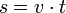dengan arti dan satuan dalam SI:

• s = jarak tempuh (m)

• v = kecepatan (m/s)

• t = waktu (s)

### [sunting]Gerak lurus berubah beraturan

Gerak lurus berubah beraturan (GLBB) adalah gerak lurus suatu obyek, di mana kecepatannya berubah terhadap waktu akibat adanya percepatan yang tetap. Akibat adanya percepatan rumus jarak yang ditempuh tidak lagi linier melainkan kuadratik.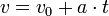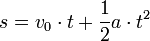dengan arti dan satuan dalam SI:

• v0 = kecepatan mula-mula (m/s)

• a = percepatan (m/s2)

• t = waktu (s)

• s = Jarak tempuh/perpindahan (m)

# 2. MOTION IN A STRAIGHT LINE

In mechanics we are interested in trying to understand the motion of objects. In this chapter, the motion of objects in 1 dimension will be discussed. Motion in 1 dimension is motion along a straight line.

## 2.1. Position

The position of an object along a straight line can be uniquely identified by its distance from a (user chosen) origin. (see Figure 2.1). Note: the position is fully specified by 1 coordinate (that is why this a 1 dimensional problem).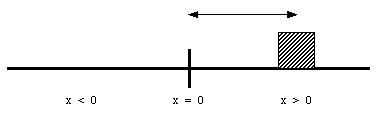Figure 2.1. One-dimensional position.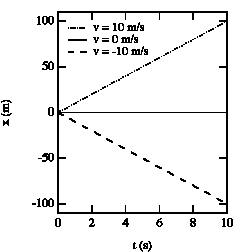Figure 2.2. x vs. t graphs for various velocities.

For a given problem, the origin can be chosen at whatever point is convenient. For example, the position of the object at time t = 0 is often chosen as the origin. The position of the object will in general be a function of time: x(t). Figure 2.2. shows the position as a function of time for an object at rest, and for objects moving to the left and to the right.

The slope of the curve in the position versus time graph depends on the velocity of the object. See for example Figure 2.3. After 10 seconds, the cheetah has covered a distance of 310 meter, the human 100 meter, and the pig 50 meter. Obviously, the cheetah has the highest velocity. A similar conclusion is obtained when we consider the time required to cover a fixed distance. The cheetah covers 300 meter in 10 s, the human in 30 s, and the pig requires 60 s. It is clear that a steeper slope of the curve in the x vs. t graph corresponds to a higher velocity.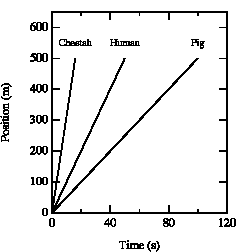Figure 2.3. x vs. t graphs for various creatures.

## 2.2. Velocity

An object that changes its position has a non-zero velocity. The average velocityof an object during a specified time interval is defined as: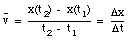If the object moves to the right, the average velocity is positive. An object moving to the left has a negative average velocity. It is clear from the definition of the average velocity thatdepends only on the position of the object at time t = t1 and at time t = t2. This is nicely illustrated in sample problem 2-1 and 2-2.

Sample Problem 2-1

You drive a beat-up pickup truck down a straight road for 5.2 mi at 43 mi/h, at which point you run out of fuel. You walk 1.2 mi farther, to the nearest gas station, in 27 min (= 0.450 h). What is your average velocity from the time you started your truck to the time that you arrived at the station ?

The pickup truck initially covers a distance of 5.2 miles with a velocity of 43 miles/hour. This takes 7.3 minutes. After the pickup truck runs out of gas, it takes you 27 minutes to walk to the nearest gas station which is 1.2 miles down the road. When you arrive at the gas station, you have covered (5.2 + 1.2) = 6.4 miles, during a period of (7.3 + 27) = 34.3 minutes. Your average velocity up to this point is: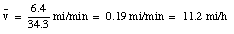Sample Problem 2-2

Suppose you next carry the fuel back to the truck, making the round-trip in 35 min. What is your average velocity for the full journey, from the start of your driving to you arrival back at the truck with the fuel ?

It takes you another 35 minutes to walk back to your car. When you reach your truck, you are again 5.2 miles from the origin, and have been traveling for (34.4 + 35) = 69.4 minutes. At that point your average velocity is: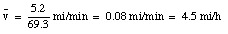After this episode, you return back home. You cover the 5.2 miles again in 7.3 minutes (velocity equals 43 miles/hour). When you arrives home, you are 0 miles from your origin, and obviously your average velocity is: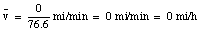The average velocity of the pickup truck which was left in the garage is also 0 miles/hour. Since the average velocity of an object depends only on its initial and final location and time, and not on the motion of the object in between, it is in general not a useful parameter. A more useful quantity is the instantaneous velocity of an object at a given instant. The instantaneous velocity is the value that the average velocity approaches as the time interval over which it is measured approaches zero: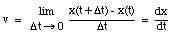For example: see sample problem 2-5.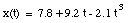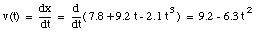The velocity of the object at t = 3.5 s can now be calculated: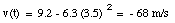## 2.3. Acceleration

The velocity of an object is defined in terms of the change of position of that object over time. A quantity used to describe the change of the velocity of an object over time is the acceleration a. The average acceleration over a time interval between t1 and t2 is defined as: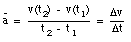Note the similarity between the definition of the average velocity and the definition of the average acceleration. The instantaneous acceleration a is defined as: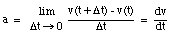From the definition of the acceleration, it is clear that the acceleration has the following units: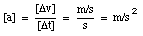A positive acceleration is in general interpreted as meaning an increase in velocity. However, this is not correct. From the definition of the acceleration, we can conclude that the acceleration is positive if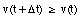This is obviously true if the velocities are positive, and the velocity is increasing with time. However, it is also true for negative velocities if the velocity becomes less negative over time.

## 2.4. Constant Acceleration

Objects falling under the influence of gravity are one example of objects moving with constant acceleration. A constant acceleration means that the acceleration does not depend on time: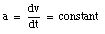Integrating this equation, the velocity of the object can be obtained: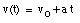where v0 is the velocity of the object at time t = 0. From the velocity, the position of the object as function of time can be calculated: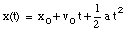where x0 is the position of the object at time t = 0.

Note 1: verify these relations by integrating the formulas for the position and the velocity.

Note 2: the equations of motion are the basis for most problems (see sample problem 7).

Sample Problem 2-8

Spotting a police car, you brake a Porsche from 75 km/h to 45 km/h over a distance of 88m. a) What is the acceleration, assumed to be constant ? b) What is the elapsed time ? c) If you continue to slow down with the acceleration calculated in (a) above, how much time would elapse in bringing the car to rest from 75 km/h ? d) In (c) above, what distance would be covered ? e) Suppose that, on a second trial with the acceleration calculated in (a) above and a different initial velocity, you bring your car to rest after traversing 200 m. What was the total braking time ?

a) Our starting points are the equations of motion:(1)(2)

The following information is provided:

* v(t = 0) = v0 = 75 km/h = 20.8 m/s

* v(t1) = 45 km/h = 12.5 m/s

* x(t = 0) = x0 = 0 m (Note: origin defined as position of Porsche at t = 0 s)

* x(t1) = 88 m

* a = constant

From eq.(1) we obtain: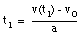(3)

Substitute (3) in (2):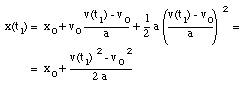(4)

From eq.(4) we can obtain the acceleration a: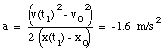(5)

b) Substitute eq.(5) into eq.(3):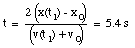(6)

c) The car is at rest at time t2: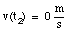(7)

Substituting the acceleration calculated using eq.(5) into eq.(3):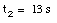(8)

d) Substitute t2 (from eq.(8)) and a (from eq.(5)) into eq.(2):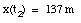(9)

e) The following information is provided:

* v(t3) = 0 m/s (Note: Porsche at rest at t = t3)

* x(t = 0) = x0 = 0 m (Note: origin defined as position of Porsche at t = 0)

* x(t3) = 200 m

* a = constant = – 1.6 m/s2

Eq.(1) tells us: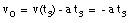(10)

Substitute eq.(10) into eq.(2):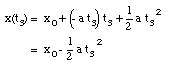(11)

The time t3 can now easily be calculated: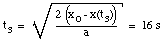(12)

## 2.5. Gravitational Acceleration

A special case of constant acceleration is free fall (falling in vacuum). In problems of free fall, the direction of free fall is defined along the y-axis, and the positive position along the y-axis corresponds to upward motion. The acceleration due to gravity (g) equals 9.8 m/s2 (along the negative y-axis). The equations of motion for free fall are very similar to those discussed previously for constant acceleration: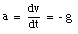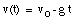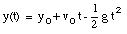where y0 and v0 are the position and the velocity of the object at time t = 0.

Example

A pitcher tosses a baseball straight up, with an initial speed of 25 m/s. (a) How long does it take to reach its highest point ? (b) How high does the ball rise above its release point ? (c) How long will it take for the ball to reach a point 25 m above its release point.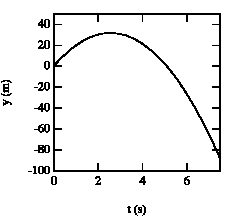Figure 2.4. Vertical position of baseball as function of time.

a) Our starting points are the equations of motion:The initial conditions are:

* v(t = 0) = v0 = 25 m/s (upwards movement)

* y(t = 0) = y0 = 0 m (Note: origin defined as position of ball at t = 0)

* g = 9.8 m/s2

The highest point is obtained at time t = t1. At that point, the velocity is zero: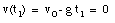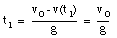The ball reaches its highest point after 2.6 s (see Figure 2.4).

b) The position of the ball at t1 = 2.6 s can be easily calculated: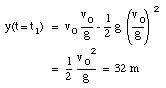c) The quation for y(t) can be easily rewritten as: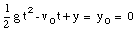where y is the height of the ball at time t. This Equation can be easily solved for t: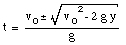Using the initial conditions specified in (a) this equation can be used to calculate the time at which the ball reaches a height of 25 m (y = 25 m):

t = 1.4 s

t = 3.7 s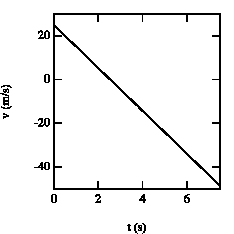Figure 2.5. Velocity of the baseball as function of time.

The velocities of the ball at these times are (see also Figure 2.5):

v(t = 1.4 s) = + 11.3 m/s

v(t = 3.7 s) = – 11.3 m/s

At t = 1.4 s, the ball is at y = 25 m with positive velocity (upwards motion). At t = 2.6 s, the ball reaches its highest point (v = 0). After t = 2.6 s, the ball starts falling down (negative velocity). At t= 3.7 s the ball is located again at y = 25 m, but now moves downwards.

## Basic Definitions

### Position

First, we will talk about one of the most basic concepts of physics, position. There are many ways to express the position of a point particle, one of the simplest and most well known way being the Cartesian Coordinate System. In a Cartesian Coordinate System, the position of a particle is expressed as an ordered pair, the x-coordinate, a y-coordinate in a two or three dimensional space, and a z-coordinate in a three dimensional space.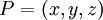The point (0, 0, 0) is commonly known as the origin. The x, y, and z axis pass through the origin at right angles to create the foundation for point-plotting.

### Displacement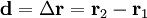### Velocity

The velocity is defined to be the rate of change of position with respect to time. For now, this basic definition should hold. Just knowing the basic definition of velocity should be enough to answer many of the problems we will investigate later. The SI unit for velocity is the meter/second. This should not surprise you as the SI unit for length is the meter and the SI unit for time is the second.

Since velocity is the rate of change of position, one can conclude that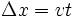And since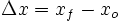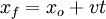We have just derived a basic formula to calculate the position of an object given the initial position, a constant velocity, and the time elapsed.

There is a significant difference between velocity and speed. Velocity is a vector – It has two pieces of information: a magnitude and a direction. Speed is the magnitude of velocity, a scalar value. An important example of this distinction is centripetal acceleration. Since the definition of acceleration is a change in velocity, there can be a change in speed, direction or both. In centripetal acceleration, the speed of a particle in uniform circular motion (such as a weight attached to a string swung above one’s head) does not have to change, though since its direction is constantly changing it experiences acceleration.

#### Examples

1. If point x has an initial position at 0, Answer: To solve this problem, simply use the formula we derived earlier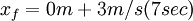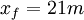### Speed

Speed is the magnitude of velocity. It is a scalar.

### Acceleration

Acceleration is the rate of change of velocity. It is the derivative of velocity over time, as well as the second derivative of position over time.

### Momentum

Momentum is mass times velocity. Momentum is always conserved, this is known as Conservation of Momentum.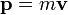### Force

According to Newton’s Second Law of Motion, force is mass times acceleration. Force is commonly confused with momentum. The SI unit of force is the newton.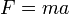Suppose a car with a mass of 200kg is accelerating at 5 m/s/s. Since F = ma, you can calculate the force to be 1000 Newtons

However, if same car had a constant velocity of 5 m/s, the force is 0N because the car is not accelerating.

## Simple Forms of Motion

### Constant Velocity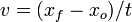### Constant Acceleration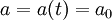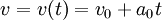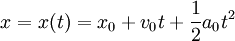#Gerak Lurus Beraturan (GLB)

• Friday Aug 8,2008 10:02 AM

• By sanSuatu benda dikatakan melakukan gerak lurus beraturan jika kecepatannya selalu konstan. Kecepatan konstan artinya besar kecepatan alias kelajuan dan arah kecepatan selalu konstan. Karena besar kecepatan alias kelajuan dan arah kecepatan selalu konstan maka bisa dikatakan bahwa benda bergerak pada lintasan lurus dengan kelajuan konstan.

Misalnya sebuah mobil bergerak lurus ke arah timur dengan kelajuan konstan 10 m/s. Ini berarti mobil bergerak lurus ke arah timur sejauh 10 meter setiap sekon. Karena kelajuannya konstan maka setelah 2 sekon, mobil bergerak lurus ke arah timur sejauh 20 meter, setelah 3 sekon mobil bergerak lurus ke arah timur sejauh 30 meter dan seterusnya   bandingkan dengan gambar di samping. Perhatikan besar dan arah panah. Panjang panah mewakili besar kecepatan alias kelajuan, sedangkan arah panah mewakili arah kecepatan. Arah kecepatan mobil = arah perpindahan mobil = arah gerak mobil.

Pada umumaya GLB didasari oleh Hukum Newton I (  F = 0 ).

 S = X = v . t ; a = v/t = dv/dt = 0 v = S/t = ds/dt = tetap

Tanda  (selisih) menyatakan nilai rata-rata.

Tanda d (diferensial) menyatakan nilai sesaat.

Kinematika adalah cabang fisika yang mempelajari gerak dengan menghiraukan penyebab gerak. Mengenai penyebab gerak akan dibahas dalam Dinamika.

Soal-Solusi GLB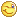Faiz mengendarai sepeda motor dengan kelajuan tetap 36 km/jam selama 30 menit. Tentukan jarak tempuh Faiz dalam satuan meter !

Diketahui

V = 36 km/jam

=  10 m/s (lihat konversi diatas)

t  = 30 menit  karena 1 menit = 60 sekon

= 30 (60 sekon)

= 1800 sekon

Ditanyakan

X =  meter

Jawab

Rumus

X  = V x t

= 10 m/s x 1800 s

= 18.000 meter